Numerical Question Type

# What is a Numerical Question?In a Numerical Question type, students are presented with a quantitative problem or equation that they will solve and enter a number-based answer. This question type is static: every student will receive an identical question and the correct answers for all students will therefore be identical.

To create a question wherein every student receives different starting numbers and will have different correct answers, choose the Calculated or Simple Calculated Question Type instead.

Numerical Questions allow students to demonstrate lower-order learning objectives, according to Bloom’s taxonomy including such action verbs as solve, calculate, apply, complete, etc.

# Add a Simple Calculate Question

1. Find the Administration block and under Question bank click Questions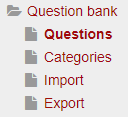1. Select the desired Category for the new question, or add a New Category.  The default Category is named “Default for…” followed by the name of the course.1. Click Create a new question button.1. Select the Question TypeNumerical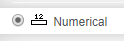1. Click the Add button to start creating the question content.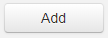# Settings: General

1. In the Category field, select the desired Category for the Question (if not already done during the Add a Numerical Question step).

2. In the Question Name field, enter the desired name for the Question.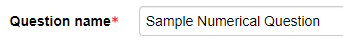1. In the Question Text field, type in the content of the question.

• This field is HTML-editable, so it can contain images, special characters, links to outside content, etc.

•  Entry blanks can appear in the body of the text or separate from the text.

• The question text in the example is “What is 4.1 + 1.1?”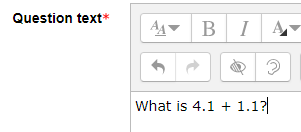1. For the Default Mark field, it is recommended to leave this at the default value “1”. The point value of the question within the quiz can be altered within the Quiz Activity.1. The General Feedback field can be filled in with feedback that all students will receive after completing this question.

1. Fill in the Answer 1 formula field with the correct numerical answer for this question.

Answer 1 in the example, 5.2, is the numerical answer to the example question: “What is 4.1 + 1.1?”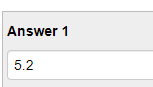1. The next field is the Error field, even though the actual text is entered on the next line.

• Error allows students to receive full credit for answers that are plus or minus the amount entered.

• For the example, if the correct answer is 5.2, and the Error is set to 0.1, then the answers 5.1, 5.2, and 5.3 would all be accepted for full credit.

This is because the values 5.1 and 5.3 are obtained when subtracting the Error value from 5.2 or adding the Error value to 5.2.

• Enter an Error of zero (0) if students must enter the exact correct answer. The example image has an Answer 1 value of 5.2 and the Error value of 0.1. In the Grade field, since this is the correct answer, choose 100%.1. In the Feedback field, enter any feedback that student should receive if they submit the correct answer.

2. Click on Blanks for 3 more answers to generate:

• Answers from partially correct calculations. The Grade can be changed to a percentage other than 100%. Feedback can be included to explain why this answer only earned partial credit.

• Answers from specific incorrect calculations. The Grade can be changed to a “None”. Feedback can be included to explain why this answer earned no credit.

# Settings: Unit Handling and Units

While Moodle claims to allow students to enter units, the functionality is not robust.

# Settings: Multiple Tries

If Adaptive Mode is used in the settings on a Quiz, point deductions and feedback for each try can be entered here. View the Multiple Tries Settings Document for more detailed instructions.

# Save and Preview

• Once all the fields are complete and there are no more errors, click on the blue Save changes button to be redirected to the Question Bank screen. The new question will appear highlighted in blue and/or green.

• Previewing each new question to confirm that it is working properly is recommended. The Magnifying Glass Icon next to the question name allows a quick preview of that question outside of a Quiz Activity.